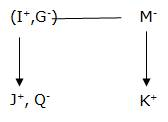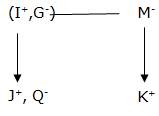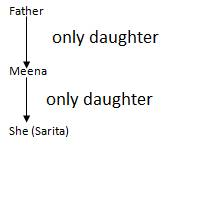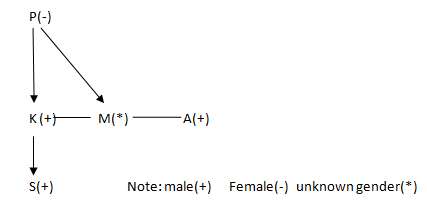## Blood Relations – Practice set 2

1. If X + Y means X is the daughter of Y; X – Y means X is the brother of Y; X % Y means X is the father of Y and X x Y means X is the sister of Y. Which of the following means I is the niece of J?

a) J – N % C x I

b) I x C – N % J

c) J + M x C % I

d) I x C + N – J

e) None of these

d) I x C + N – J

D

Explanation

d

I x C → I is the sister of C

C + N → C is the daughter of N

and N – J → N is the brother of I.

Hence, I is niece of J.

2. M is the father of N who is the son of V. In order to know the relation of M to P, which of the statement/statements is/are necessary?

1. P is the brother of V.

2. The daughter of N is the granddaughter of V.

a) Only (1)

b) Only (2)

c) Either (1) or (2)

d) (1) and (2) both

e) None of these

a) Only (1)

A

Explanation

a

M is the father of N and N is the son of V.

Hence, V is the mother of N.

From (1), P is the brother of V

Therefore, M is the brother-in-law of P because V is the wife of M.

From (2), the daughter of N, is the granddaughter of V. From this we do not get any relation of M to P.

3. A is the son of C; C and Q are sisters; Z is the mother of Q and P is the son of Z. Which of the following statements is true?

a) P and A are cousins

b) P is the maternal uncle of A

c) Q is the maternal grandfather of A

d) C and P are sisters

e) None of these

b) P is the maternal uncle of A

B

Explanation

b

C and Q are sisters and A is the son of C. Hence, C is the mother of A or Z is the mother Q.

Hence, Z is the maternal grandmother of A. P is the son of Z. Hence, P is the maternal uncle of A.

4. If P + Q means P is the brother of Q; P x Q means P is the wife of Q and P % Q means P is the daughter of Q then which of the following means D is the uncle of A?

a) A % B x C + D

b) A x B + C % D

c) A + C % B x D

d) C % B x D + A

e) None of these

e) None of these

E

Explanation

e

4. Anupam said to a lady sitting in a car, “The only daughter of the brother of my wife is the sister-in-law of the brother of your sister.” How the husband of the lady is related to Anupam?

a) Maternal uncle

b) Uncle

c) Father

d) Son-in-law

e) None of these

d) Son-in-law

D

Explanation

d

Anupam’s son-in-law is the brother of the lady who was sitting in the car. Hence, the husband is also the son-in-law of Anupam.

D.5-6) I is the father of J, M is mother of K and sister of G G is mother of J There are three females in the family Q is sister of J

5. How is K related to Q?

a) Cousin (sister)

b) Cousin (brother)

c) Sister-in-law

d) Brother

e) None of these

b) Cousin (brother)

B

Explanation

b6. How is I related to M?

a) Brother

b) Father

c) Sister

d) Brother-in-law

e) None of these

d) Brother-in-law

D

Explanation

d7. Introducing Sarita, Meena said, “She is the only daughter of my father’s only daughter.” How is Meena related to Sarita ?

a) Niece

b) Mother

c) Aunt

d) Cousin

e) None of these

b) Mother

B

Explanation

bP # Q’ means ‘P is the daughter of Q’

P © Q’ means ‘Q is the brother of P’

P = Q’ means ‘Q is the sister of P’

P & Q’ means ‘P is the son of Q’

P * Q’ means ‘P is the father of Q’

P @ Q’ means ‘P is the mother of Q’

8. What does the expression ‘A @ M = L © D &N’?

a) N is the wife of A

b) M is the son of N

c) M is the daughter of N

d) N is the husband of A

e) None of these

d) N is the husband of A

D

Explanation

d

9. Which of the following indicates that ‘A is the paternal uncle of S’?

a) A & M # P @ K © S

b) A & M & K @ P © S

c) S & K & P @ M © A

d) d) S & P # M @ K © A

e) None of these

c) S & K & P @ M © A

C

Explanation

c10. Pointing to a photograph. Balu said, “He is the son of the only daughter of the father of my brother.” How Balu is related to the man in the photograph?

a) Nephew

b) Brother

c) Father

d) Maternal Uncle

e) None of these